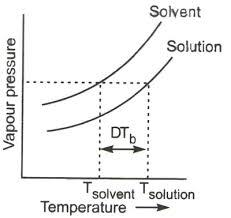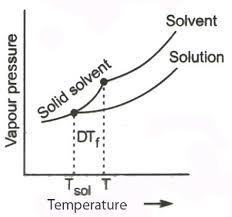QuestionAnswers

# When common salt is dissolved in water(A) The melting point of solution increases(B) The boiling point of solution decreases(C) Both melting and boiling point decrease(D) The boiling point of solution increases.

Hint: Salt is a non- volatile solute which when added to water will reduce the vapour pressure of the solution. Hence, in order to make the solution boil, we need to give more heat. Then only the vapour pressure of the solution will become equal to the atmospheric pressure and hence it can boil.

- Common salt is a non-volatile solute here and water is the solvent.
- In options, there are mainly two terms , melting point and boiling point, so firstly we can have a look at what is this melting and boiling point.
- Melting point is defined as the temperature at which the solid changes into liquid at atmospheric pressure. At this temperature, the solid and liquid, both phases are in equilibrium with each other. For Eg : Melting point of ice is ${ 0 }^{ 0 }C$ .
- Boiling point is defined as the temperature at which the vapour pressure of the liquid becomes equal to atmospheric pressure. At this point, both the liquid and gaseous phases are in equilibrium with each other. For Eg : Boiling point of water is ${ 100 }^{ 0 }C$.
- So, when salt (non- volatile solute) is added to a water (solution), the vapour pressure of the solution is lowered, it is because of the fact that now the surface of the water is not only filled with water molecules but also with the salt molecules.
- Since, the vapour pressure of the solution is lowered now, it can boil only at a raised temperature, then only the required vapour pressure will become equal to the atmospheric pressure. And this is known as ‘elevation of boiling point’.Above given is the graph of elevation of boiling point
- So, when common salt is added to water, the boiling point of the solution increases.
- And when considering the melting point, when a non volatile solute is added to a solvent, the melting point of the solution decreases and this phenomenon is referred to as the ‘depression in melting point’.Above given is the graph of depression in melting point.
- Here, in this question, there is no option available as decrease in melting point and increase in boiling point as both.and so only one option is available before us. i.e, the increase in boiling point.
Therefore, the correct option is option (D) The boiling point of solution increases.

Note: There are four colligative properties in total - lowering of vapour pressure, depression in freezing point, elevation of boiling point and osmotic pressure. Of which lowering of vapour pressure, depression in freezing point, elevation of boiling point are applicable when a non volatile solute is added to a solvent.